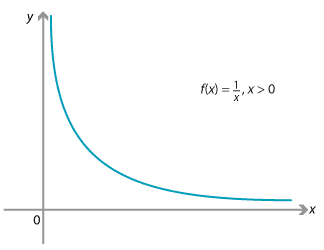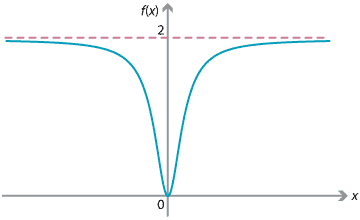## Content

### Limit of a function at infinity

Just as we examined the limit of a sequence, we can apply the same idea to examine the behaviour of a function $$f(x)$$ as $$x$$ becomes very large.

For example, the following diagram shows the graph of $$f(x)=\dfrac{1}{x}$$, for $$x > 0$$. The value of the function $$f(x)$$ becomes very small as $$x$$ becomes very large.We write

$\lim_{x\to \infty} \dfrac{1}{x} = 0.$

One of the steps involved in sketching the graph of a function is to consider the behaviour of the function for large values of $$x$$. This will be covered in the module Applications of differentiation.

The following graph is of the function $$f(x) = \dfrac{2x^2}{1+x^2}$$. We can see that, as $$x$$ becomes very large, the graph levels out and approaches, but does not reach, a height of 2.We can analyse this behaviour in terms of limits. Using the idea we saw in the section Limit of a sequence, we divide the numerator and denominator by $$x^2$$:

$\lim_{x\to \infty} \dfrac{2x^2}{1+x^2} = \lim_{x\to \infty} \dfrac{2}{\dfrac{1}{x^2}+1} = 2.$

Note that as $$x$$ goes to negative infinity we obtain the same limit. That is,

$\lim_{x\to -\infty} \dfrac{2x^2}{1+x^2} = 2.$

This means that the function approaches, but does not reach, the value 2 as $$x$$ becomes very large. The line $$y=2$$ is called a horizontal asymptote for the function.

Exercise 3

Find the horizontal asymptote for the function $$f(x) = \dfrac{x^2-1}{3x^2+1}$$.

Examining the long-term behaviour of a function is a very important idea. For example, an object moving up and down under gravity on a spring, taking account of the inelasticity of the spring, is sometimes referred to as damped simple harmonic motion. The displacement, $$x(t)$$, of the object from the centre of motion at time $$t$$ can be shown to have the form

$x(t) = Ae^{-\alpha t} \sin \beta t,$

where $$A$$, $$\alpha$$ and $$\beta$$ are positive constants. The factor $$Ae^{-\alpha t}$$ gives the amplitude of the motion. As $$t$$ increases, this factor $$Ae^{-\alpha t}$$ diminishes, as we would expect. Since the factor $$\sin \beta t$$ remains bounded, we can write

$\lim_{t\to \infty} x(t) = \lim_{t\to \infty} Ae^{-\alpha t} \sin \beta t = 0.$

In the long term, the object returns to its original position.

Next page - Content - Limit at a point